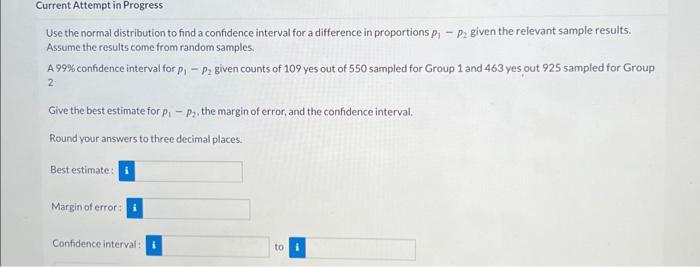Home / Expert Answers / Statistics and Probability / use-the-normal-distribution-to-find-a-confidence-interval-for-a-difference-in-proportions-p1p-pa255

# (Solved): Use the normal distribution to find a confidence interval for a difference in proportions p1p ...Use the normal distribution to find a confidence interval for a difference in proportions given the relevant sample results. Assume the results come from random samples, A confidence interval for given counts of 109 yes out of 550 sampled for Group 1 and 463 yes out 925 sampled for Group 2. Give the best estimate for , the margin of error, and the confidence interval. Round your answers to three decimal places. Bestestimate: Margin of error: Confidence interval: to

We have an Answer from Expert